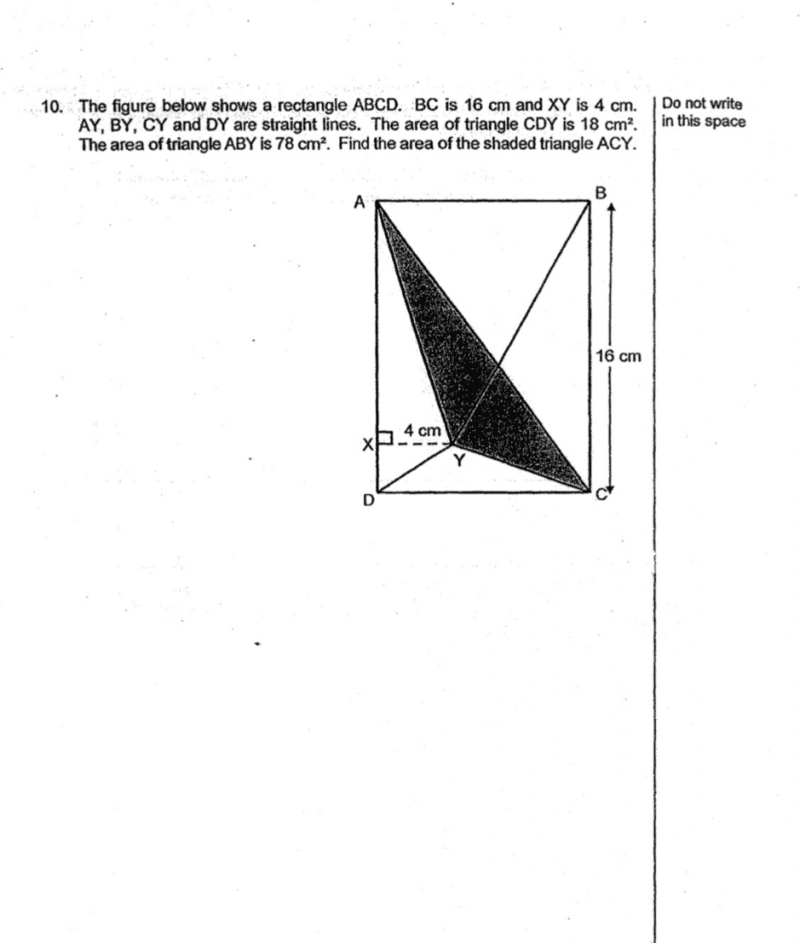# QuestionThing to note for student: Area of ABY + area of CDY = half of area of rectangle ABCD.

Why? Bcos heights of both triangles exactly make up the height (16cm) of rectangle.

And base of triangles = base of rectangle.

Hence, half area of rectangle, ie Triangle ACD = 18+78

= 96 cm2

Area of triangle ADY = 0.5 x 16 x 4 = 32 cm2

Therefore, area of shaded region = 96 – 32 – 18

= 46 cm2

Hmm, tricky question.  I try.

The important thing is to try to find the width of the rectangle CD.  Let this be b.

Let h be the height of triangle CDY.

So area of triangle CDY is 0.5bh = 18
=> (1) b = 36/h

Also, area of triangle ABY is 0.5b(16-h) = 78
=> (2) b = 156/(16-h)

Combining (1) and (2),

36/h = 156/(16-h)
36(16-h) = 156h
576 – 36h = 156h
192h = 576
=> h = 3

Therefore, b = 36/h = 36/3 = 12

Area of triangle ADY = 0.5(16)(4) = 32

So, area of shaded triangle ACY
= Area of triangle ACD – Area of triangle ADY – Area of triangle CDY
= 0.5(12)(16) – 32 – 18
= 96 – 32 -18
= 46 cm2 #

0 Replies 1 Like